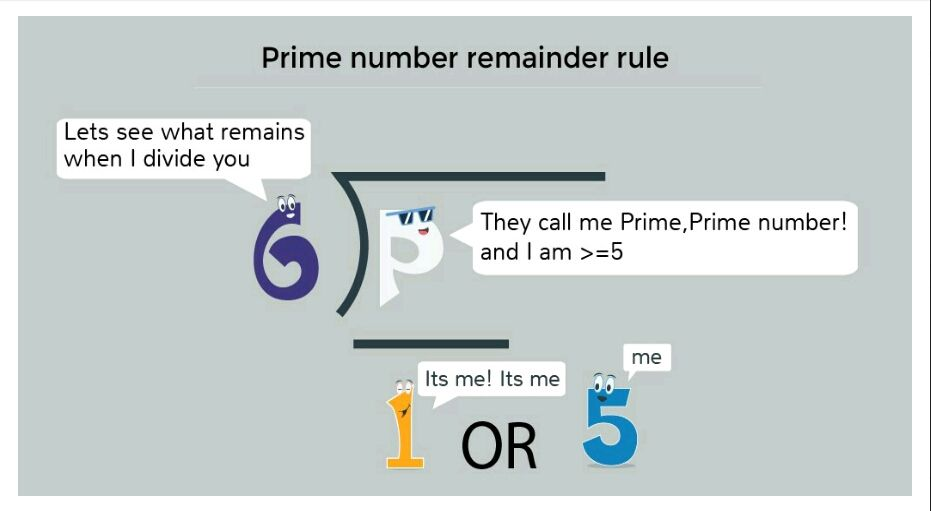# prime number remainder rule

in #steemeducation4 years ago# Rule

The remainder when a prime number p >= 5 is divided by 6 is either 1 or 5. This trick can be used to quickly check the possibility of a number being prime.

However, if a number on being divided by 6 gives a remainder of 1 or 5, the number need not necessarily be prime.

# Examples

11 and 17 both are prime numbers and leave remainder 5 when divided by 6.

13 and 19 both are prime numbers and leave remainder 1 when divided by 6.

However, 25 when divided by 6 also leaves a remainder of 1 but 25 is not a prime number.
So, this rule could be used only to check a quick possibility of a number being prime.

STEEM 0.68
TRX 0.10
JST 0.076
BTC 58250.79
ETH 4638.80
BNB 627.82
SBD 7.31